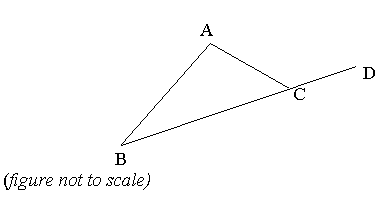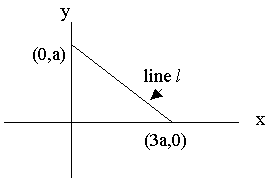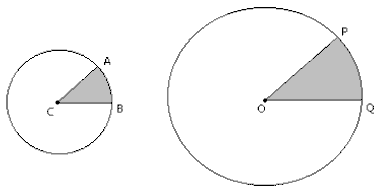50万+已关注

# SAT数学练习题整理8

2019-02-06 17:26:00来源：网络

新东方在线SAT频道为大家带来SAT数学练习题整理8一文，希望对大家SAT备考有所帮助。更多精彩尽请关注新东方在线SAT频道!

1. 3x + y = 19 , and x + 3y = 1.

Find the value of 2x + 2y

A. 20

B. 18

C. 11

D. 10

E. 5

2. The price of a cycle is reduced by 25 per cent. The new price is reduced by a further 20 per cent. The two reductions together are equal to a single reduction of

A. 45%

B. 40%

C. 35%

D. 32.5%

E. 30%

3. x and y are integers

x + y < 11 , and x > 6

What is the smallest possible value of x - y ?

A. 1

B. 2

C. 4

D. -2

E. -4

4. If x5y4z2 <0 , which of the following must be true?

I xy <0

II yz <0

III xz <0

A. I

B. II

C. III

D. I and II

E. None5. BCD is a line segment and Angle BAC = Angle ACB ; Angle ACD = ?

A. 140

B. 100

C. 120

D. 60

E. it cannot be determined from the information given

6. Which of the following integers is in the solution set of │1 – 3x│ < 5 ?

I -1

II 1

III 2

A. I only

B. II only

C. III only

D. I and II only

E. I, II and III

7. In a certain village, m litres of water are required per household per month. At this rate, if there are n households in the village, how long (in months) will p litres of water last?

A. p /mn

B. mn / p

C. mp / n

D. np / m

E. npm8. In the figure below, what is the slope of line l ?

A. - 3

B. - 1/3

C. 0

D. 1/3

E. 3

9. What digit appears in the units place in the number obtained when 2320 is multiplied out?

A. 0

B. 2

C. 4

D. 6

E. 810. Radius of circle center O is 3 times the radius of circle center C.

Angle ACB = Angle POQ

If the shaded area of circle C is 2 then what is the area of the shaded part of circle O ?

A. 6

B. 12

C. 18

D. 36

E. 3/2

Explanation:

To solve a pair of simultaneous equations such as those given we can add or subtract them.

Adding we get 4x + 4y = 20

Therefore 2x + 2y = 10

Explanation:

Let the original price of the cycle be 100. After the first reduction the price will be 75.

This new price is then reduced by 20% = 0.8 x 75 = 60

60 represents a reduction of 40 percent on the original.

Explanation:

The smallest value will be obtained by giving x the smallest possible value while y gets the largest possible value. The smallest value for x = 7. This means that y will have to be less than or equal to 3 (so that x + y <11), but we want the largest value of y which is 3.

The difference, x - y will be 4.

Explanation:

The value of the expression must be negative. But squares and even powers are always positive, and so y and z could be either negative or positive. Therefore x5 must be negative, and x is negative.

However, each of the choices given has either a y or a z in it, so e cannot be certain whether the product is negative or positive.

Explanation:

If a value x is selected for angle ACB and one quarter of the value is taken for angle BAC, we could work out the value for the other angle of the triangle. Also angle ACD would be 180 - x. The problem is that we could choose a range of different values for x and still make them fit the given information. Therefore we cannot work out any one value for angle ACD.

Explanation:

Remember the sign │x│ means the absolute value of x (the distance between the number and zero on the number line). Absolute values are always positive.

You can try them out and find that –1 and 0 both give values less than 5 for the expression │1 – 3x│, but with 2 you get 1-6 = -5 giving an absolute value of 5.

In fact there are only three integers in the solution set of this expression: -1, 0 and 1

Explanation:

Pick sensible numbers and work the problem out. Then go back to letters.

So if 100 liters are needed per month, 20 households will need 100 x 20 liters per month.

1000 liters will last 1000/ 100 x 20 months. Putting the letters back = p/mn

Explanation:

The slope is the y side of the triangle divided by the x side of the triangle (y2 - y1) / (x2 - x1)

This is a / 3a which is the equivalent of 1/3 but the slope is negative (because the line slopes down from left to right as we look at it). Hence -1/3

Explanation:

Do not attempt to work this out. Look for the pattern in the last digits in all the powers of 2.

The series starts out 2...4...8...16...32....64...128..256...

Already the pattern is clear; the last digits repeat in a pattern 2,4,8,6,2,4,8,6, etc.

Every power that is a multiple of 4 will end in a 6 . 320 is a multiple of 4.

Explanation:

If the radii of the two circle are in the ratio 1 : 3, then the areas of the circle will be in the ratio (1)2 : (3)2which is 1 : 9

The marked areas represent equivalent fractions of the areas since the angles at the centers are the same. Hence the areas of the shaded parts are also in the ratio 1 : 9.

If the area of the smaller is 2, then the area of the larger will be 18.

以上就为大家整理的“SAT数学练习题整理8”，更多精彩内容，请关注新东方在线SAT频道。

SATII精讲班
SAT名师一对一

SAT考生必修课
SAT考生，高效提分，安心之选

• 课时 : 91琚晋蓉张霄

￥2680

18人 已报到

免费试听

• 1
• 2
• 3
• 4
• 5
• 6
• 7
• 8
• 9
• 10